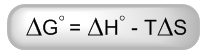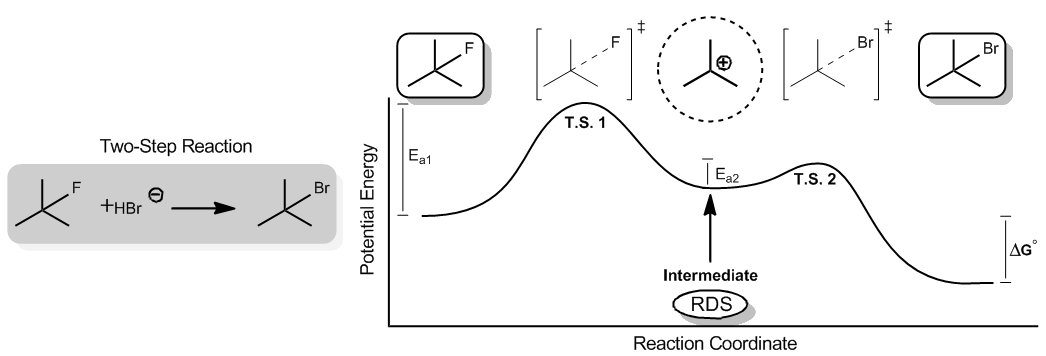Start typing, then use the up and down aroows to select an option from the list.

# Organic Chemistry

Learn the toughest concepts covered in Organic Chemistry with step-by-step video tutorials and practice problems by world-class tutors.

6. Thermodynamics and Kinetics

Time for Gibbs Free Energy, the most important equation for understanding reaction favorability! It’s going to be important that we understand the significance of all the terms in this equation.1

### concept

Breaking down the different terms of the Gibbs Free Energy equation.5m
Play a video:
0

1. Enthalpy (ΔH°) is the sum of bond dissociation energies for the reaction.

• Negative values (-) indicate the making of new bonds = Exothermic
• Positive values (+) indicate the breaking of new bonds = Endothermic

2. Entropy (ΔS) is the tendency of a system to take its most probable form.

• Negative values (-) indicate less probable = Unfavored
• Positive values (+) indicate more probable = Favored

3. Temperature (T) amplifies the effect of entropy on the overall favorability.

2

### concept

Intermediates vs. Transition States6m
Play a video: